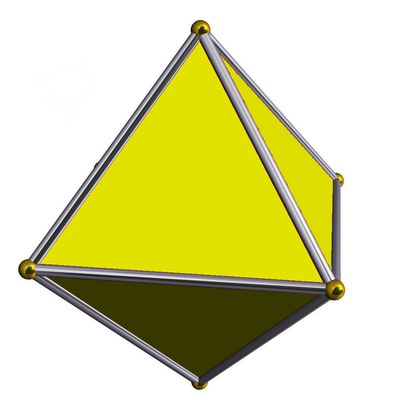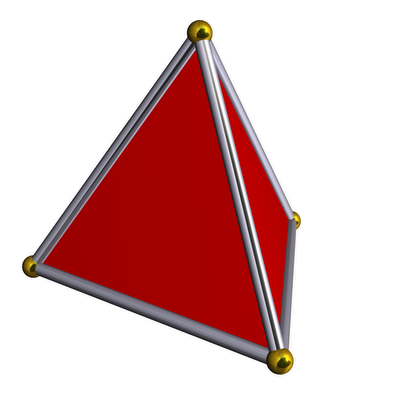Integral Octonions (Part 11)

## Integral Octonions (Part 11)

#### John Baez and Greg Egan

Take a bunch of equal-sized solid balls in 8 dimensions. Pick one... and then get as many others to touch it as you can.

You can get 240 balls to touch it — no more. And unlike in 3 dimensions, there's no 'wiggle room': you're forced into a specific arrangement of balls, apart from your ability to rotate the whole configuration.

You can continue packing balls so that each ball touches 240 others — and unlike in 3 dimensions, there's no choice about how to do it: their centers are forced to lie at a lattice of points called the E8 lattice.

If we pick a point in this lattice, it has 240 nearest neighbors. Let's call these the first shell. It has 2160 second-nearest neighbors. Let's call these the second shell.

And here's what fascinates me now. You can take the first shell, rotate it, and expand it so that the resulting 240 points form a subset of the second shell!

In fact, there are 270 different subsets of this type. And if you pick two of them that happen to be disjoint, you can use them to create a copy of the Leech lattice inside $$\mathrm{E}_8 \oplus \mathrm{E}_8 \oplus \mathrm{E}_8$$ — that is, the direct sum of three copies of the $$\mathrm{E}_8$$ lattice!

There are exactly 17,280 ways to pick two disjoint subsets of this type. And each way gives a distinct embedding of the Leech lattice in $$\mathrm{E}_8 \oplus \mathrm{E}_8 \oplus \mathrm{E}_8$$.

We're seeing some big numbers here! Some seem harder to understand than others. Though it's not easy to prove that the $$\mathrm{E}_8$$ lattice gives the largest number of balls that can touch a given central one in 8 dimensions, it's easy to check by hand that it has 240 points in the first shell, and 2160 in the second shell. This is old, well-known stuff.

Greg Egan discovered the other two numbers using computer calculations. By now we understand one of them fairly well: we found a simple construction that gives 270 subsets of the second shell that are rotated, rescaled versions of the first shell. The construction is nice, because it involves the Fano plane: the projective plane over the field with 2 elements! So, I'll explain that today.

The number 17,280 remains mysterious.

### Why 270?

I want to show you that there are at least 270 subsets of the second shell of the $$\mathrm{E}_8$$ lattice that are rotated, rescaled copies of the first shell. In fact that's the exact number — but I only know that because I trust Egan's computing skills. We did, however, figure out a very pretty proof that there are at least 270. Here are the key steps:

1. There's a rotational symmetry of the $$\mathrm{E}_8$$ lattice mapping any point of the first shell to any point to any other point, and similarly for the second shell.

2. There are 240 points in the first shell.

3. There are 2160 points in the second shell.

4. There are 30 rotated, expanded copies of the first shell containing your favorite point in the second shell.

Item 1 lets us exploit symmetry. Since your favorite point in the second shell is just like any other point, we can construct 30 × 2160 rotated, expanded copies of the first shell in the second shell. But these copies are not all distinct: if we counted them by naive multiplication, we would be overcounting by a factor of 240, since each has 240 points. So, the correct count is

$$30 \times 2160 / 240 = 30 \times 9 = 270$$

as desired.

Why are items 1-4 true?

In Part 4 and Part 5 of these series we saw that the points in the first shell are the vertices of a highly symmetrical polytope, the E8 root polytope. We saw that this polytope has 240 vertices, 2160 7-orthoplex faces, and 17,280 7-simplex faces. Remember, an n-orthoplex is the $$n$$-dimensional generalization of an octahedron:while an n-simplex is the $$n$$-dimensional generalization of a tetrahedron:General facts about Coxeter groups, reviewed in Part 5, imply that the symmetries of the $$\mathrm{E}_8$$ root polytope act transitively on its vertices, its 7-orthoplex faces and its 7-simplex faces. We also saw that each 7-orthoplex face has a vector in the second shell as its outward-pointing normal. Thus there are 2160 points in the second shell, and the symmetries act transitively on these too. This takes care of items 1-3.

So, the only really new fact is item 4: there are 30 rotated, expanded copies of the first shell containing a chosen point in the second shell! This is what I want to show. More precisely, I'll show that there are at least 30. So far I only know there are at most 30 thanks to Egan's computer search. We can probably dream up a proof, but we haven't gotten around to it.

### Why 30?

Remember the standard description of the $$\mathrm{E}_8$$ lattice. Define a half-integer to be an integer plus $$\frac{1}{2}$$. The $$\mathrm{E}_8$$ lattice consists of all 8-tuples of real numbers

$$(x_0, x_1, x_2, x_3, x_4, x_5, x_6, x_7)$$

such that

• the $$x_i$$ are either all integers or all half-integers, and

• the sum of all the $$x_i$$ is even.

The shortest nonzero vectors in this lattice — the vectors in the first shell — have length $$\sqrt{2}$$, like this:

$$(1,1,0,0,0,0,0,0)$$

The second shortest nonzero vectors — the vectors in the second shell — have length $$2$$, like this:

$$(1,1,1,1,0,0,0,0)$$

So, we have the opportunity to find an expanded copy of $$\mathrm{E}_8$$ lattice, dilated by a factor of $$\sqrt{2}$$, inside the $$\mathrm{E}_8$$ lattice.

Our goal is to find 30 such copies containing our favorite point in the second shell. Let's take this as our favorite:

$$(2,0,0,0,0,0,0,0)$$

And here's the cool part: there's one such copy for each way to give the set

$$\{1,2,3,4,5,6,7\}$$

the structure of a projective plane!

Up to isomorphism there's just one projective plane of order 7: the projective plane over the field of 2 elements, called the Fano plane. It has 7 points and 7 lines, 3 points on each line and 3 lines through each point:

It's a projective plane in the axiomatic sense: each pair of distinct points lies on a unique line, and each pair of distinct lines intersects in a unique point.

So, how many projective plane structures are there on the set

$$\{1,2,3,4,5,6,7\} ?$$

We get one from any way of labelling the diagram above by numbers from 1 to 7. There are $$7!$$ of these labellings. However, not every one gives a distinct Fano plane structure, since the Fano plane has symmetries. Its symmetry group is famous: it's the second smallest nonabelian simple group, and it has 168 elements. So, we get

$$\frac{7!}{168} = \frac{1 \cdot 2 \cdot 3 \cdot 4 \cdot 5 \cdot 6 \cdot 7}{24 \cdot 7} = 30$$

different Fano plane structures on the set

$$\{1,2,3,4,5,6,7\}$$

How can we use one of these Fano plane structures to find an expanded copy of the $$\mathrm{E}_8$$ lattice inside the $$\mathrm{E}_8$$ lattice?

Let $$e_i$$ be the $$i$$th basis vector of $$\mathbb{R}^8$$, where $$i = 0,1,2,3,4,5,6,7$$. We form the lattice consisting of all integer linear combinations of:

• the vectors $$2e_i$$,

• the vectors

$$\pm e_0 \pm e_i \pm e_j \pm e_k$$ where $$i,j,k$$ lie on some line in the Fano plane,

• the vectors

$$\pm e_p \pm e_q \pm e_r \pm e_s$$ where $$p,q,r,s$$ all lie off some line in the Fano plane.

Pondering the discussion of 'Kirmse integers' in Part 6, you'll see this lattice is a rotated copy of the $$\mathrm{E}_8$$ lattice, rescaled by a factor of $$\sqrt{2}$$. However, you can also immediately see that it's a sublattice of the $$\mathrm{E}_8$$ lattice as defined above!

With a bit more work, you can see that each different projective plane structure on $$\{1,2,3,4,5,6,7\}$$ gives a different lattice of this sort. So, we get 30 of them.

Since each such lattice consists of linear combinations of its shortest vectors, we get 30 distinct subsets of the second shell that are rotated, rescaled copies of the first shell. Moreover, all these contain our favorite point in the second shell,

$$2e_0 = (2,0,0,0,0,0,0,0)$$

### Why 17,280?

So, I've shown how to construct at least 270 subsets of the second shell of the $$\mathrm{E}_8$$ lattice that are rotated, rescaled copies of the first shell. And in fact, that's all there are.

I said any way to pick two of these 270 subsets that are disjoint gives a copy of the Leech lattice inside $$\mathrm{E}_8 \oplus \mathrm{E}_8 \oplus \mathrm{E}_8$$. Egan explained how this works in Part 9.

But why are there 17,280 ways to choose two disjoint subsets of this sort? We don't have a proof yet; his computer calculations just reveal that it's true. Since

$$270 \times 64 = 3^3 \times 10 \times 4^3 = 12^3 \times 10 = 17,280$$

it would suffice to check that for each of our 270 subsets we can find 64 others that are disjoint from it. And indeed, his computer calculations verify this, and have told us a lot about what these 64 are like. But we haven't yet turned this into a human-readable proof that there are 64.

Another thing he checked that each of these 17,280 disjoint pairs gives a distinct sublattice of $$\mathrm{E}_8 \oplus \mathrm{E}_8 \oplus \mathrm{E}_8$$. So, this procedure gives 17,280 distinct copies of the Leech lattice sitting in here. But again, this deserves a nice proof.

By the way: it's not as if we're short of Leech lattices sitting inside $$\mathrm{E}_8 \oplus \mathrm{E}_8 \oplus \mathrm{E}_8$$. In Part 10 we saw, without any computer assistance, that there are at least 244,035,421. However, the ones obtained by the construction we're discussing now are especially nice: for example, we've seen they give Jordan subrings of the exceptional Jordan algebra. So I think these are worth understanding in more detail.

And besides, they're leading us into fun questions about the geometry of the $$\mathrm{E}_8$$ lattice! Any excuse for thinking about $$\mathrm{E}_8$$ is a good thing as far as I'm concerned. It's endlessly rewarding in its beauty: today we've seen how the Fano plane is hiding inside it, in a rather abstract way. Of course, we already knew the Fano plane was involved in defining octonion multiplication — but nothing today required octonion multiplication. To define the product of octonions we need to orient the lines of the Fano plane, breaking its symmetry group — but today we didn't need to do this!

You can also read comments on the n-Category Café, and make your own comments or ask questions there!

© 2014 John Baez
baez@math.removethis.ucr.andthis.edu
home